Study S3 Physics Light - Reflection - Geniebook# Light - Reflection

In this article, we will be going through the topic of Reflection as per the requirements of Secondary 3 Physics in Singapore. We will cover the following subtopics.

• Terms and conventions used in Reflection
• Laws of Reflection
• Ray Diagram
• Characteristics of a plane mirror image

## Characteristics Of Plane Mirror Image

The plane mirror image is (as compared to the object)

• Laterally inverted
• Same size
• Upright / erect
• Same distance away from the mirror
• Virtual - cannot be formed on the screen

## Reflection Diagram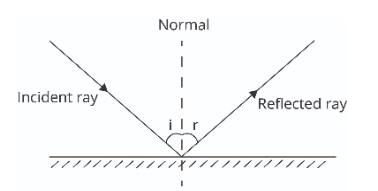An incident ray is a ray of light that strikes a surface.

The ray of light that bounces back from the surface is called the reflected ray

Angle of Incidence, i, is the angle made by the incident ray with the normal.

Angle of reflection, r, is the angle made by the reflected ray with the normal.

Normal is a line perpendicular to the surface. It divides the angle formed between the incident and the reflected ray into two equal angles.

## Laws Of Reflection

• ### First Law Of Reflection

The incident ray, the reflected ray and the normal at the point of incidence, all lie in the same plane.

• ### Second Law Of Reflection

The angle of incidence ( i ), is equal to the angle of reflection ( r ).

### Practice Questions

Question 1:

Which of the following diagrams is correct?Solution:

Option 4 is the correct answer

Option 1 and Option 3 are wrong as the direction of the incident rays and the reflected rays is not correct.

Option 2 is not correct as it does not follow the second law of reflection i.e the angle of incidence is equal to the angle of reflection.

Question 2:

A light ray is incident on the plane mirror as shown.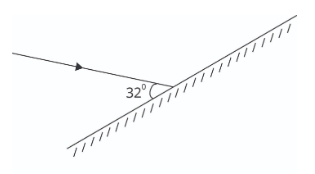What is the angle of reflection?

1. 32o
2. 58o
3. 116o
4. 148o

Solution:

Option B is the correct answer

Explaination:

The angle of incidence is measured from the incident ray with the normal.
The angle of incidence\begin{align}\\[2ex] &= 90^{\circ } - 32^{\circ}\\[2ex] &= 58^{\circ } \end{align}

From the second law of reflection,

Angle of incidence $$=$$ angle of reflection

Thus, the angle of reflection in the above diagram is also 58o.

## Ray Diagram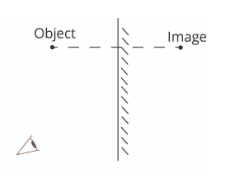Step 1:

Locate the image behind the mirror. The distance of the object to the mirror is equal to the distance of the image to the mirror.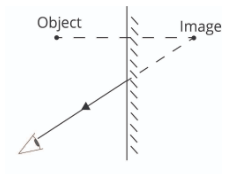Step 2:

Draw the light ray from the image to the eye. The line behind the mirror is dotted to denote that it is an imaginary line. We draw arrows only on the solid ray.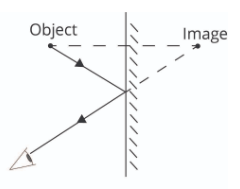Step 3:

Draw the incident ray from the object to the point of incidence on the mirror and mark the arrows accordingly.

Question 3:

Which of the following diagrams is correct?Solution:

Option 1 is the correct answer

Image formed must be directly on the opposite side of the mirror.

Example 1:

In the diagram below, the boy wishes to see his entire body in the mirror.What is the minimum length of the mirror to allow him to do so?

Solution:By the laws of reflection and similar triangles, the minimum length of the mirror needed is half of his height.
Length\begin{align}\\[2ex] &= \frac{1}{2} \times 140\\[2ex] &= 70 \text{ cm} \end{align}

## Types Of Reflection

### Regular ReflectionRegular reflection occurs at the surface of a plane like a plain mirror. Reflected rays after regular reflection are parallel.

The normal drawn at the point of incidence are all parallel to each other.

### Irregular ReflectionWhen a parallel light beam reaches a rough unpolished surface, the light rays are reflected in various directions, or the light rays are dispersed. This is called irregular reflection.

The normal drawn at the point of incidence are all in different directions and hence the reflected rays are also in different directions. Thus, the reflected rays are dispersed.

## Conclusion

In this article, we discussed the topic of Reflection in Plain Mirrors. Even though you would have learnt this topic in previously, in this article we have focussed on the following subtopics:

• Terms and conventions used in Reflection
• Laws of Reflection
• Ray Diagram
• Characteristics of a plain mirror image

Continue Learning
Light - Reflection Units and Measurements
Kinematics - Distance & Displacement Dynamics
Mass, Weight & Density Thermal Properties Of Matter
Moments Temperature
Kinetic Model of Matter Waves
Work, Power & Energy Pressure
Transfer Of Thermal EnergyPrimaryPrimary 1Primary 2Primary 3Primary 4Primary 5Primary 6SecondarySecondary 1Secondary 2Secondary 3Physics
Light - Reflection
Units And Measurements
Kinematics - Distance & Displacement
Dynamics
Mass, Weight & Density
Thermal Properties Of Matter
Moments
Temperature
Kinetic Model of Matter
Waves
Work, Power & Energy
Pressure
Transfer Of Thermal EnergyChemistryMathsSecondary 4